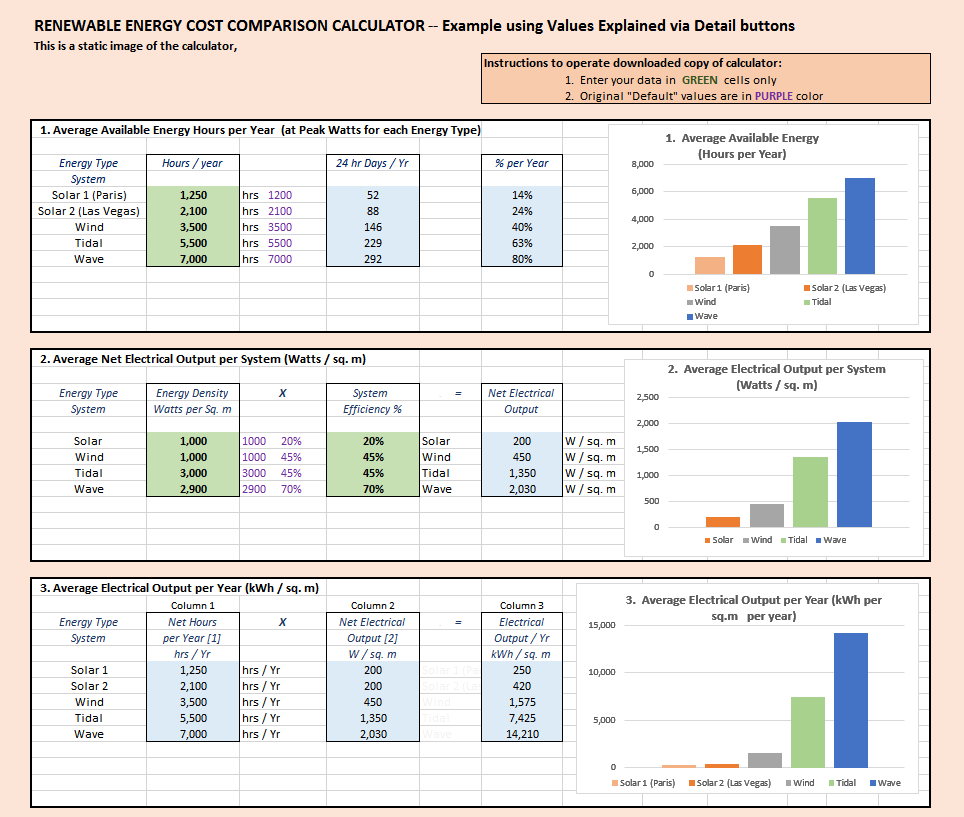This data is compiled from the previous 2 sections

Click X  to close this note:

Solar Power Density & Energy Conversion Efficiency     Assumptions

"If the extraterrestrial solar radiation is 1367 watts per square meter (the value when the
Earth–Sun distance is 1 astronomical unit), then the direct sunlight at Earth's surface
when the Sun is at the zenith is about 1050 W/m2, but the total amount (direct and indirect
from the atmosphere) hitting the ground is around 1120 W/m2."

The Average Solar Power Density for PV solar panels is considered to be 1,000 W /sq. m

http://www.solstats.com/blog/solar-energy/what-is-solar-peak-power/

PV Panel Energy Conversion Efficiency:
The Shockley-Queisser limit for the efficiency of a single-junction solar cell under unconcentrated sunlight is 34%

"There are a few solar panels available that are exceeding 19% efficiency."

Average solar power in compared to electrical energy out = 20% Efficiency  (2015)

Hence, the solar radiation converted by a PV Solar Panel is (1000 W / sq. m X 20%) = 200 Watts per sq. m

2010+     2015           2016+        Comparisons